## Summary

Natural Language Understanding (NLU) is an important field of Natural Language Processing, which enables several
applications ranging from question answering to fully automated conversational agents. In practice, the NLU is the first
step of a conversational agent that processes the user’s raw utterance. It typically comprises both Slot Filling
and Intent Classification tasks in order to create a structured representation of the utterance.
Intent classification aims to predict the intent of the utterance, while slot filling focuses on the extraction of its semantic concepts.

This blog provides a deep-dive analysis of state-of-the-art NLU models, by including two main groups of NLU models, namely:

• Joint Intent Prediction & Slot filling NLU, where intent classification and slot filling are jointly optimized and learned. Here, we have analyzed both the JointBERT and Stack-propagation NLU models.

• Context-aware NLU that, differently from JointBERT and Stack-propagation, take as input not only the current user utterance but also the previous utterances as context. Here, we have analyzed both BERT-DST and SimpleTOD models. Although the original implementation of both BERT-DST and SimpleTOD models do not perform intent prediction, they can be easily extended for joint intent classification and slot filling.

As the vast majority of the analyzed NLU models rely on the BERT architecture, this blog post starts by introducing the BERT model.
Then, after describing all the considered NLU models, this blog ends with a comparative analysis between them.

## BERT background

BERT’s model architecture is a multi-layer bidirectional Transformer encoder based on the original Transformer model. Accordingly, it consists of a stack of multiple identical blocks each containing a multi-head self-attention and a fully connected sub-layer with residual connections.

### Input & Output Representations

The input to BERT is a sequence of tokens (words), and the output is a sequence of vectors, one for each input token. To make BERT handle a variety of downstream tasks, the input representation of BERT is able to unambiguously represent both a single sentence and a pair of sentences in one token sequence (see Figure 1) .

The first token of every sequence is always a special classification token [CLS], whose final hidden state is used as the aggregate sequence representation. The final hidden states of the other tokens are used as token-level representations.

For sentence pairs tasks (e.g., Q&A), sentence pairs are packed together into a single sequence, where tokens from the two input sentences are separated by another special token - [SEP]. Additionally, BERT’s input layer adds an additional segment embedding to differentiate tokens from the pair of sentences. For single text sentence tasks (e.g., sentiment classification), the input sequence also ends with the special [SEP] token.

Therefore, the input representation of a given token is constructed by summing the corresponding token, segment, and position embeddings (see Figure 2).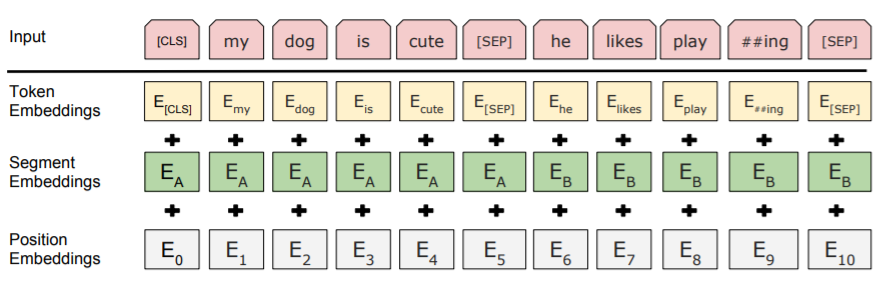Figure 2: BERT input representation. The input embeddings are the sum of the token embeddings, the segmentation embeddings, and the position embeddings (image from ).

### Pre-training BERT

To learn bidirectional contextualized representations and inter-sentence relationships, the BERT model is pre-trained
on two unsupervised language modeling tasks, using the BooksCorpus and the English Wikipedia corpora, namely:

by using the special [MASK] token, in the input sequence;

2. Next Sentence Prediction (NSP): where the objective is to predict whether two input segments follow each other in
the original text. Positive examples are created by taking consecutive sentences from the text corpus,
whereas negative examples are created by picking segments from different documents.

The pre-trained BERT model provides powerful context-dependent sentence-level and token-level representations,
which can be used for a wide range of target-specific tasks (e.g., NER, Q&A, etc - see Figure 3), without substantial
task-specific architecture modifications (i.e. with just extra projection layers and simple fine-tuning procedures).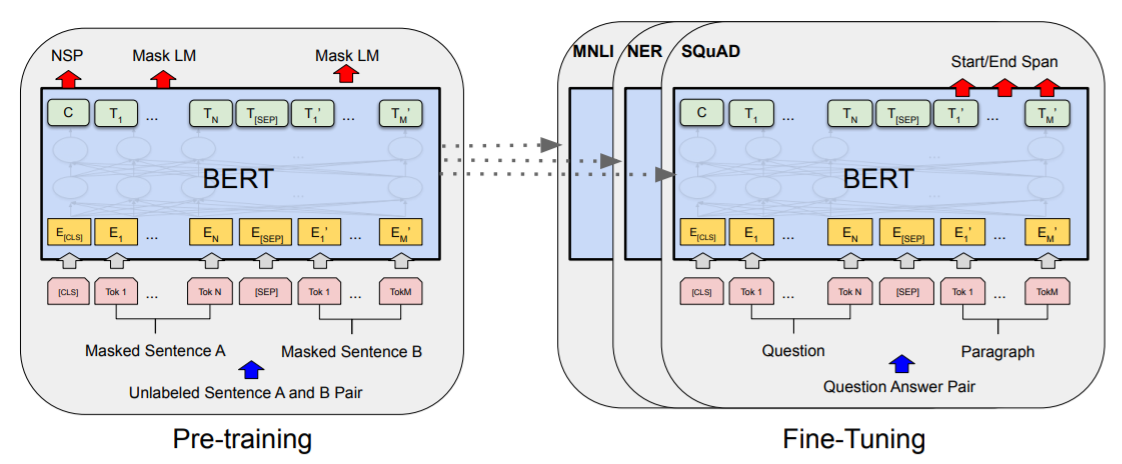Figure 3: Overall pre-training and fine-tuning procedures for BERT (image from ).

As described next, BERT can be easily extended to joint intent classification and slot filling (e.g., JointBERT) or
dialogue state tracking (e.g., BERT-DST).

## Joint Intent prediction & Slot filling NLU

This section describes both JointBERT and Stack-propagation NLU models. These models follow the principle of jointly
learn intent prediction and slot filling tasks, in order to explicitly explore the correlations between intent and slots.

Nevertheless, as described in the following subsections, while JointBERT follows a muti-task learning approach,
Stack-propagation NLU follows a stack-propagation framework. The former learns correlations between the two tasks
(i.e., intent prediction and slot filling) by a shared encoder. The latter directly uses the intent as input for slot filling.

### JointBERT: BERT for Joint Intent Classification and Slot Filling

As illustrated in Figure 4 , JointBERT extends the conventional BERT architecture to a joint intent classification
and slot filling.

#### Encoding module

The ultimate goal of JointBERT is to map from a single user utterance to the intent and slots. Therefore, the input user utterance is represented as a sequence of tokens in the BERT’s input format:

[CLS] → User utterance tokens → [SEP]

So, given an input token sequence $[x_0, x_1, …, x_N]$, it outputs a sequence of latent representations
$[h_0, h_1, …, h_N]$ for each token. 

#### Joint Intent Classification and Slot Filling

JointBERT simultaneously performs intent classification and slot filling.

For intent prediction , it simply uses the latent representation of the [CLS] token as input ($h_0$) to a softmax classifier:
$y^{i}=\text{softmax}(W^ih_0+b^i)$.

For slot filling, the latent representations of the other tokens $[h_1, …, h_N]$ are fed to a softmax classifier
to classify over the slot filling labels: $y^{s}_n=\text{softmax}(W^sh_n+b^s), n\in 1, …, N$.

The learning objective is to maximize the conditional probability $p(y^i, y^s|x)$, which can be achieved by
minimizing the cross-entropy loss. 

#### Conditional Random Field

Since slot label predictions are dependent on predictions for surrounding words, the authors investigated the impact of adding CRF for modeling slot label dependencies (on top of the joint BERT model). In practice, this is achieved by replacing the softmax classifier with a CRF.

Experimental results suggest that JointBERT with CRF performs comparably to JointBERT, probably due to the self-attention mechanism in Transformer, which may have sufficiently modeled the label structures.

As expected, experimental results suggest that without joint intent and slot filling learning there is a drop in both intent classification and slot filling $F_1$. 

### Stack-Propagation: A Stack-Propagation Framework with Token-Level Intent Detection for Spoken Language Understanding

Similar to JointBERT, the Stack-Propagation NLU model also performs a joint intent
classification and slot filling. However, while JointBERT follows a multi-task learning framework,
in which correlations between these two tasks are learned by a shared encoder, Stack-Propagation SLU follows a
stack-propagation framework (see Figure 5). The idea is to directly use the intent as input for slot filling as
a way to capture the intent semantic knowledge. 

#### Stack-Propagation framework

As illustrated in Figure 6, the architecture of the stack-propagation model comprises an encoder and two decoders.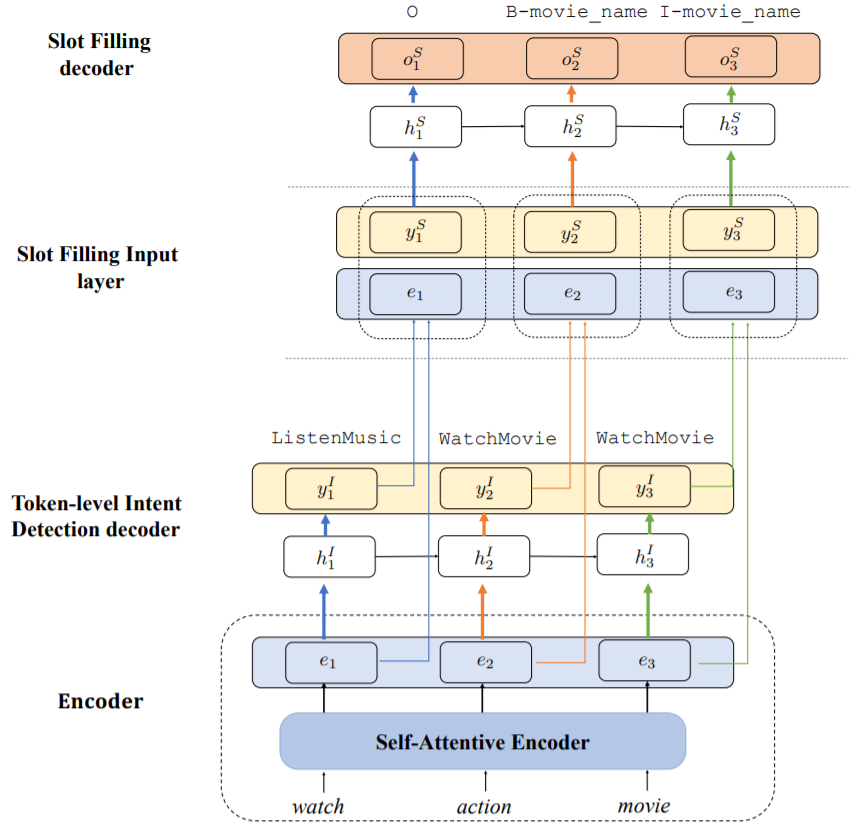Figure 6: Stack-Propagation framework for joint intent detection and slot filling (image from ).
• Self-Attentive Encoder: it maps from the tokenized user utterance $[x_1, x_2, …, x_N]$ to contextual hidden
presentations $[e_1, e_2, …, e_N]$. To do so, it comprises a BiLSTM with a self-attention mechanism to leverage both
advantages of temporal features and contextual information. Note that the authors assessed the effect of BERT,
by replacing their self-attentive encoder with a BERT-base model. Experimental results demonstrated the benefits of
using a strong pre-trained model like BERT for NLU tasks.

• Token-Level Intent Detection Decoder: it consists of a unidirectional LSTM that performs a token-level intent
detection. They argue that token-level intent detection may reduce error propagation when compared to sentence-level
intent detection. At each decoding step $i$, the decoder state $h_i^I$ is calculated from the
previous decoder state $h_{i-1}^I$, the previous emitted intent label distribution $y_{i-1}^I$ and
the aligned encoder hidden state $e_i$: $h_i^I=f(h_{i-1}^I, y_{i-1}^I, e_i)$.

• Stack-propagation for Slot Filling (Decoder): the input for slot filling consists of a concatenation of the intent
output distribution $y_{i}^I$ and the aligned encoder hidden state $e_i$. Similar to the intent decoder,
the slot filling decoder also consists of a unidirectional LSTM.

Note that both intent detection and slot filling are optimized simultaneously via a joint learning scheme. 

## Context-aware NLU

This section describes both BERT-DST and SimpleTOD models that consider the dialogue context for NLU purposes.
However, while BERT-DST takes the current user utterance and the previous system utterance as input,
SimpleTOD considers all the previous user and system utterances as input context.

Additionally, BERT-DST makes use of softmax classifiers on top of BERT for slot filling,
whereas SimpleTOD uses a unidirectional Transformer decoder architecture (i.e., GPT-2) and
recasts slot filling and state tracking in a generative fashion.

### BERT-DST: Scalable End-to-End Dialogue State Tracking with Bidirectional Encoder Representations from Transformer

The architecture of the BERT-DST framework is illustrated in Figure 7.
For each user turn, BERT-DST takes as input the recent dialogue context,
which comprises the system utterance in the previous turn and the current user utterance,
and outputs a turn-level level dialogue state (i.e. per-slot span values).
This is accomplished with the following major components:

1. Dialogue Context Encoding Module : first, the dialogue context input (previous system utterance + current user utterance) is encoded by the BERT-based encoding module to produce contextualized sentence-level and token-level representations.

2. Classification Module : then, the sentence-level hidden representation (i.e. the hidden representation of the [CLS] token) is used to generate a categorical distribution over three types of slot values: none , don’t care, or a span.

3. Per-slot Span Prediction Module : it gathers the token-level representations and outputs the slot value’s start and end positions.

4. Dialogue State Update Mechanism : finally, a rule-based update mechanism is used to track dialogue states across turns. 

#### Dialogue Context Encoding Module

Since the dialogue encoding module is based on BERT, the dialogue context input is represented as a token sequence in
the BERT’s input format:

[CLS] → System utterance tokens → [SEP] → User utterance tokens → [SEP]

Considering $[x_0, x_1, …, x_n]$ as the tokenized input sequence, BERT’s input layer embeds each token $x_{0}$ into
an embedding $e_{i}$ - which is the sum of the token, segment, and position embeddings.

Then, the embedded input sequence $[e_0, e_1, …, e_n]$ is passed through the BERT’s encoder in order to output the hidden representations
of each input token $[t_0, t_1, …, t_n]$. As described in the following subsections, the sentence-level representation
(i.e. [CLS] token hidden state) is then used by the classification module, whereas the token-level representations $[t_1, …, t_n]$
are used by the per-slot span precision module.

It is important to note that the parameters of this module are initialized from a pre-trained BERT checkpoint and then
fine-tuned to the target-specific DST dataset. 

#### Classification Module

The classification module takes as input the sentence-level representation $t_{0}$ and aims to classify each slot $s\in S$ into one
of the following three classes {none, don’t care, span}. It simply consists of the fully connected layer with a softmax
activation function: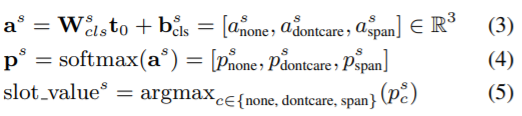It is important to note that the parameters of this module (i.e. $W^{s}_{cls}$, $b^{s}_{cls}$) are trained
from scratch with the target-specific DST dataset. 

#### Per-slot Span Prediction Module

The span prediction module takes as input the token-level representations $[t_1, …, t_n]$ and aims to predict the
span (start and end positions) of each $s\in S$.

More specifically, each token representation $t_i$ is linearly projected through a common layer,
whose output values correspond to start $\alpha_{i}^{s}$ and end $\beta_{i}^{s}$ positions.
Then, a softmax is applied to the position values to produce a probability distribution over all tokens, by which the slot value span can be determined.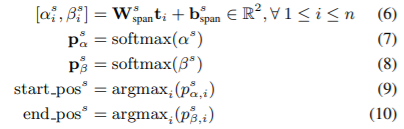It is important to note that the parameters of this module (i.e. $W^{s}_{span}$, $b^{s}_{span}$) are trained from scratch with the target-specific DST dataset. 

#### Dialogue State Update Mechanism

Dialogue state tracking is based on a rule-based update mechanism. In each turn, if the model’s turn prediction for
a slot is don’t care or a span (i.e., any value other than none), it will be used to update the dialogue state.
Otherwise, the dialogue state of the slot remains the same as the previous turn.

• Parameter Sharing: Although both classification and span prediction modules are slot-specific, the authors noticed that BERT (i.e., the dialogue context encoding module) parameters can be shared across all slots. This reduces the model parameters and transfers knowledge among slots.

• Slot Value Dropout: applied to address the under-training problem of contextual features in slot filling. That is, models tend to overfit frequent slot values in training data instead of learning contextual patterns, which reduces the performance on Out-Of-Vocabulary (OOV) slot values. To improve the performance on unseen slot values, during training, target slot values are replaced by a special [UNK] token at a certain probability.

• Span prediction loss: a weighted summation of $0.8\times\zeta_{\text{cls}}^{\text{xent}} + 0.1\times\zeta_{\text{span start}}^{\text{xent}} + 0.1\times\zeta_{\text{span end}}^{\text{xent}}$,
where $\zeta^{\text{xent}}$ corresponds to the categorical cross-entropy for the corresponding prediction target. 

### SimpleTOD: A Simple Language Model for Task-Oriented Dialogue

Typically, each component of Task-Oriented Dialogue (TOD) systems is trained independently with specific supervision.
The conventional pipeline of TOD systems usually comprises (i) Natural Language Understanding (NLU) for belief state tracking; (ii) Dialogue Policy (DP), for deciding which actions to take based on the belief state; and (iii) natural language generation (NLG) for generating responses.

SimpleTOD is a simple approach that recasts all TOD sub-tasks/components as a single sequence prediction problem by using a unidirectional (causal) pre-trained language model, i.e. the GPT-2. GPT-2 resembles the decoder from the original Transformer architecture to learn a distribution for next-word prediction.

This way, SimpleTOD can be used as a generative model for dialogue state tracking. Experiment results suggest that SimpleTOD is a robust dialogue state tracker in the presence of noisy labeled annotations. 

#### SimpleTOD overview

As illustrated in Figure 8, SimpleTOD considers all previous dialogue turns as context $C_t=[U_0, S_0, …, U_t]$,
where $U$ and $S$ denote User and System utterances, respectively; in order to generate the output sequence token by token as follows:

• It first generates the belief state $B_t=\text{SimpleTOD}(C_t)$. The belief state is a list of triplets for slots in a particular domain: (domain, slot_name, value).

• Then, the belief state is used to query a database for information. The returned rows/outputs of the database $D_t$ are then concatenated with $C_t$ and $B_t$, at the end of the output sequence, to generate the actions $A_t$ to take: $A_t=\text{SimpleTOD}(C_t, B_t, D_t)$. Actions are also generated as a list of triplets: (domain, action_type, slot_name).

• Finally, a delexicalized response $S_t$ is generated conditioned on all prior information concatenated as a single sequence: $S_t=\text{SimpleTOD}(C_t, B_t, D_t, A_t)$. The response can then be lexicalized by replacing slots and values with information from the belief state and database search results. 

Figure 9 provides a schematic representation of the different components of inputs/outputs in task-oriented dialogue.

#### Training

A single training sequence $x^t$ consists of the concatenation of context $C_t$, belief states $B_t$,
database search results $D_t$, action decisions $A_t$, and system response $S_t$, such that $x^{t}=[C_t; B_t; D_t; A_t; S_t]$.

SimpleTOD is optimized by minimizing the negative log-likelihood over the joint sequence $x^{t}$.
The output state associated with each input token is used to predict the next token - see Figure 10.

## Model’s comparison

Model Input Joint intent & slot filling DST mechanism Architecture
JointBERT  Current User Utterance Yes N/A** BERT + Softmax classifiers
Stack-propagation  Current User Utterance Yes N/A** Self-attentive encoder + 2 decoders (LSTM)
BERT-DST  Current User Utterance + Previous System Uttrance No* Rule-based BERT + Softmax classifiers
SimpleTOD  All User and System uterrances No* Directly predicts the belief state (updated slots) Unidrectional Transformer decoder (i.e. GPT-2) + Generative decoding

* Nevertheless, the architecture BERT-DST and SimpleTOD can be easily extended for joint intent prediction and slot filling.

** Although DST is not addressed in both JointBERT and Stack-propagation, a rule-based approach could be easily employed based on the extracted slots and intents.

 Jacob Devlin, Ming-Wei Chang, Kenton Lee, Kristina Toutanova, BERT: Pre-training of Deep Bidirectional Transformers for Language Understanding. NAACL-HLT (1) 2019: 4171-4186

 Chen, Qian & Zhuo, Zhu & Wang, Wen. (2019). BERT for Joint Intent Classification and Slot Filling.

 Qin, Libo & Che, Wanxiang & Li, Yangming & Wen, Haoyang & Liu, Ting. (2019). A Stack-Propagation Framework with Token-Level Intent Detection for Spoken Language Understanding. 2078-2087. 10.18653/v1/D19-1214.

 Chao, Guan-Lin & Lane, Ian. (2019). BERT-DST: Scalable End-to-End Dialogue State Tracking with Bidirectional Encoder Representations from Transformer. 1468-1472. 10.21437/Interspeech.2019-1355.

 Hosseini asl, Ehsan & McCann, Bryan & Wu, Chien-Sheng & Yavuz, Semih & Socher, Richard. (2020). A Simple Language Model for Task-Oriented Dialogue.

Blog photo by omni-chatbot.